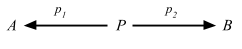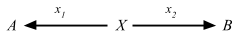### Coproduct

One of the well known duals is coproduct, which is the dual of product. Prefixing with “co-” is the convention to name duals.

Here’s the definition of products again:

Definition 2.15. In any category C, a product diagram for the objects A and B consists of an object P and arrows p1 and p2satisfying the following UMP:

Given any diagram of the formthere exists a unique u: X => P, making the diagramcommute, that is, such that x1 = p1 u and x2 = p2 u.

Flip the arrows around, and we get a coproduct diagram:Since coproducts are unique up to isomorphism, we can denote the coproduct as A + B, and [f, g] for the arrow u: A + B => X.

The “coprojections” i1: A => A + B and i2: B => A + B are usually called injections, even though they need not be “injective” in any sense.

Similar to the way products related to product type encoded as `scala.Product`, coproducts relate to the notion of sum type, or disjoint union type.

#### Algebraic datatype

First way to encode A + B might be using sealed trait and case classes.

``````sealed trait XList[A]

object XList {
case class XNil[A]() extends XList[A]
case class XCons[A](head: A, rest: XList[A]) extends XList[A]
}

XList.XCons(1, XList.XNil[Int])
// res0: XList.XCons[Int] = XCons(head = 1, rest = XNil())
``````

#### Either datatype as coproduct

If we squint `Either` can be considered a union type. We can define a type alias called `|:` for `Either` as follows:

``````type |:[+A1, +A2] = Either[A1, A2]
``````

Because Scala allows infix syntax for type constructors, we can write `Either[String, Int]` as `String |: Int`.

``````val x: String |: Int = Right(1)
// x: String |: Int = Right(value = 1)
``````

Thus far I’ve only used normal Scala features only. Cats provides a typeclass called `cats.Inject` that represents injections i1: A => A + B and i2: B => A + B. You can use it to build up a coproduct without worrying about Left or Right.

``````import cats._, cats.data._, cats.syntax.all._

val a = Inject[String, String |: Int].inj("a")
// a: String |: Int = Left(value = "a")

val one = Inject[Int, String |: Int].inj(1)
// one: String |: Int = Right(value = 1)
``````

To retrieve the value back you can call `prj`:

``````Inject[String, String |: Int].prj(a)
// res1: Option[String] = Some(value = "a")

Inject[String, String |: Int].prj(one)
// res2: Option[String] = None
``````

We can also make it look nice by using `apply` and `unapply`:

``````lazy val StringInj = Inject[String, String |: Int]

lazy val IntInj = Inject[Int, String |: Int]

val b = StringInj("b")
// b: String |: Int = Left(value = "b")

val two = IntInj(2)
// two: String |: Int = Right(value = 2)

two match {
case StringInj(x) => x
case IntInj(x)    => x.show + "!"
}
// res3: String = "2!"
``````

The reason I put colon in `|:` is to make it right-associative. This matters when you expand to three types:

``````val three = Inject[Int, String |: Int |: Boolean].inj(3)
// three: String |: Int |: Boolean = Right(value = Left(value = 3))
``````

The return type is `String |: (Int |: Boolean)`.

#### Curry-Howard encoding

An interesting read on this topic is Miles Sabin (@milessabin)’s Unboxed union types in Scala via the Curry-Howard isomorphism.

#### Shapeless.Coproduct

There’s a datatype in Cats called `EitherK[F[_], G[_], A]`, which is an either on type constructor.